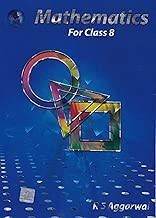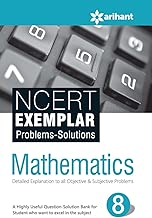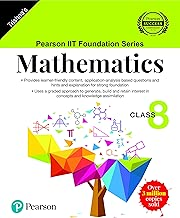# Class 8 Math### Free

Categories: Class 8

## Course Overview

### CBSE Class 8 Math Syllabus

#### Rational Numbers

•  Properties of rational numbers
• Representation of rational numbers on line number
•  Rational number between two rational numbers

#### Linear Equation in One Variable

•  Solving linear equations having variable on one side
• Application of linear equations
• Solving linear equations having variable on both sides
• Some more application of linear equations
• Reducing equations to simpler form

#### Practical Geometry

• Constructing a quadrilateral: when the length of four side and diagonal are given
• When two diagonals and three sides are given
• When two adjacent sides and three angles are known
• When three side and two included angles are given
• Some special cases : Square
• Some special cases : Rhombus

#### Data Handling

•  Looking for information
• Organizing data, Grouping data
• Circle graph or pie charts
• Chance and probability

#### Squares and Square Roots

• Square of a number
• Finding Square root of a number by repeated subtraction
• Finding Square root of a number by prime factorization method
• Finding Square root of a number by long division method
• Square root of decimals
• Estimation of square root

#### Cubes and Cube Roots

• Cubes
• Cube roots
•  Cube root of a cube number

#### Comparing Quantities

• Recalling ratio and percentage, finding the increase or decrease percentage, finding discount, Prices related to buying and selling, Sales tax/ value added tax
• Compound Interest, Deducing formula for compound interest, Rate compounded half yearly
• Application of compound interest

#### Visualizing Solid Shapes

• Views of 3D shapes
• Mapping shapes around us
• Faces, Edges and vertices

#### Exponents and Powers

• Numbers with negative and positive exponents
• Law of exponents, use of exponents to express very small and very big numbers

#### Direct and Inverse Proportions

• Direct proportion
• Inverse Proportion

#### Factorization

• What is Factorization? method of common factors Factorization by regrouping terms
•  Factorisation using identities
•  Division of algebraic expression

#### Introduction to Graphs

•  Bar graphs, pie graph and line graph
•  Linear graphs
•  Applications of linear graphs

#### Playing with Numbers

• Numbers in general form, reversing the digits of number
•  Letters for digits
• Test for divisibility by 2,3
• Test for divisibility by 5, 9, 10

### Reference Books For Class 8 MathMathematics for Class 8 by R S Aggarwal (2019-20 Session)NCERT Exemplar Problems-Solutions MATHEMATICS class 8thPearson IIT Foundation Series – Maths – Class 8